# Serial Communication between MATLAB and Arduino

Published  October 12, 2018   1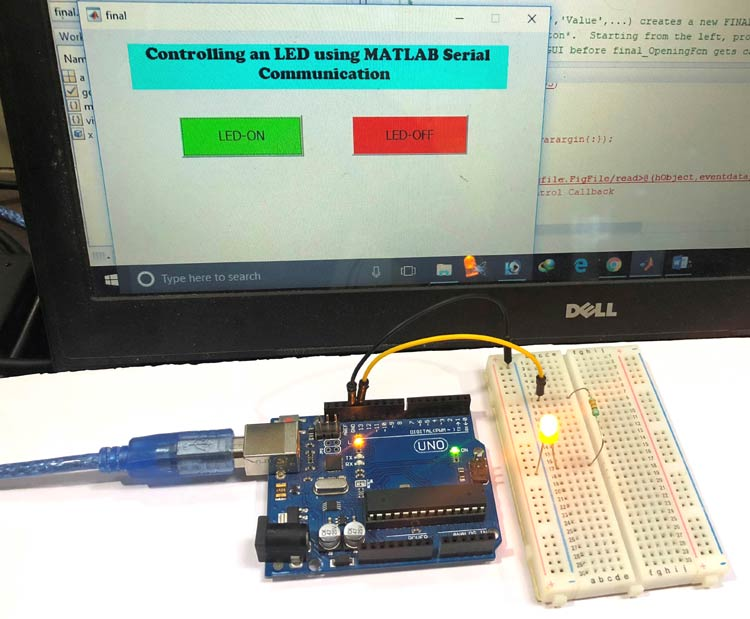MATLAB is versatile software that can be used for wide variety of applications. In previous tutorials of MATLAB, we have explained how to use MATLAB to control DC motorServo motor and Home appliances. Here in this tutorial, we will learn how to use MATLAB for Serial Communication. For the receiving end of serial communication, we are here using Arduino.

There are two ways to setup serial communication between MATLAB and Arduino, one is using command window and other is using MATLAB GUI. The Arduino code for both the methods will remain the same. If you are new to MATLAB then it is recommend to get started with simple LED blink program with MATLAB and learn the basic terminology used in MATLAB.

### Components Required

• MATLAB installed Laptop (Preference: R2016a or above versions)
• Arduino UNO
• LED (any color)
• Resistor (330 ohm)

### Circuit Diagram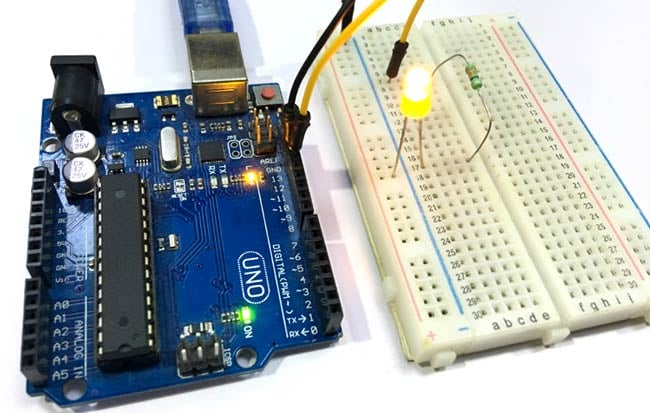The above circuit diagram will remain same for both the ways to establish serial communication between MATLAB and Arduino.

### Serial Communication using MATLAB Command Window

This is the simple method to setup serial communication between Arduino and MATLAB. Here we will simply send the data from MATLAB to the Arduino serially using command window and then Arduino read the incoming serial data. Then this serially transmitted data can be used to control anything connected to the Arduino. Here we have connected an LED to Arduino, that will be turned on and off according to the serially received data by the Arduino.

First, upload the given Arduino code in the Arduino UNO and then start coding in MATLAB Editor Window. To open a new editor script click on ‘New Script’ as shown in below image: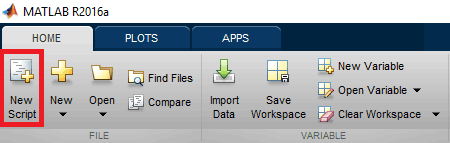Then, copy and paste the below complete MATLAB code in the editor window for serial communication between MATLAB and Arduino.

```%MATLAB Code for Serial Communication between Arduino and MATLAB

x=serial('COM18','BAUD', 9600);

fopen(x);
go = true;

while go

a= input('Press 1 to turn ON LED & 0 to turn OFF:');
fprintf(x,a);

if (a == 2)
go=false;
end
end```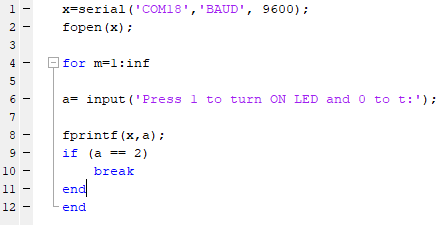In the given code, below command is used for defining the serial communication in MATLAB. Make sure the com port number is the port number on which Arduino is connected and the baud rate should be set same in the both the codes of Arduino and MATLAB.

` x=serial('COM18','BAUD', 9600);`

To open serial port use the below command,

`fopen(x);`

Below command is used to send data from MATLAB to Arduino serially, where x is for calling serial and a is the value entered by the user.

`fprintf(x,a);  `

We have use while function for creating an infinite loop and whenever the user input the number ‘2’ the loop will break.

```while go

a= input('Press 1 to turn ON LED & 0 to turn OFF:');

fprintf(x,a);
if (a == 2)
go=false;
end
end```

After completing coding the MATLAB editor script click on ‘RUN’ to run your program as shown in below image,MATLAB takes few seconds for processing the code and start the serial communication, wait until MATLAB shows ‘BUSY’ message at the bottom left corner of the software screen, as shown in below image.Now, you will see the command window for sending the user input, we have set the default message,

```'Press 1 to turn ON LED & 0 to turn OFF:'
```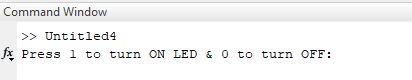Send ‘1’ to turn on the LED, ‘0’ to turn OFF the LED and ‘2’ to break the operation. You can set any number for any task, all you have to do is just change the Arduino code accordingly. Complete Arduino code is given at the end.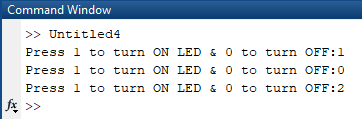You can check out the video below to understand the complete process of Sending Serial Data from MATLAB to Arduino using Command Window.

### Serial Communication using MATLAB GUI

For demonstrating the Serial Communication using MATLAB GUI, we will create two graphical buttons using MATLAB to turn on and off the LED connected to the Arduino. Data will be sent serially from MATLAB to Arduino on clicking on these buttons to turn on and off the LED. Arduino will contain the code for receiving serial data from MATLAB and controlling the LED according to serial data received. Arduino code will remain same as previous one, only difference is that, previously we were sending serial data ‘1’ and ‘0’ through command window of MATLAB, and now the same data will be sent on clinking on two graphical buttons.

To launch the GUI, type the below command in the command window

`guide`

A popup window will open, then select new blank GUI as shown in below image,Now choose two pushbuttons for turning ON and OFF the LED, as shown below,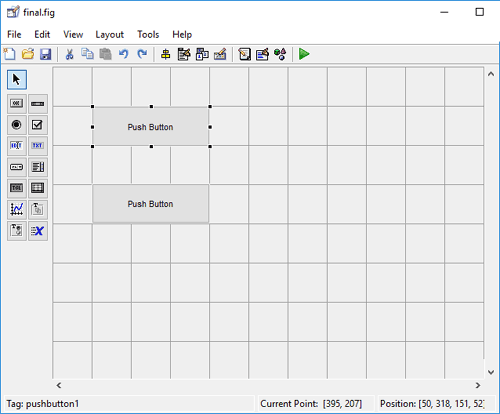To resize or to change the shape of the buttons, just click on it and you will be able to drag the corners of the button. By double-clicking on pushbutton you can change the color, string and tag of that particular button. We have customized two buttons as shown in below picture.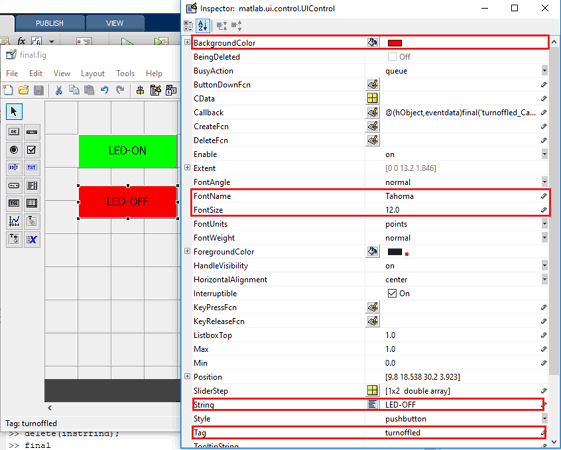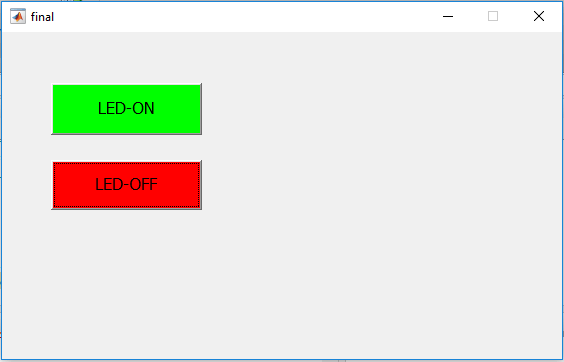You can customize the buttons as per your choice. Now when you save this, a code will generate in the Editor window of MATLAB. Edit this code according to the task you want to perform by your Arduino using the MATLAB GUI. So below we have edited the MATLAB code. You can learn more about Command window, editor window etc in Getting started with MATLAB tutorial.

Complete MATLAB code, for establishing Serial Communication between MATLAB and Arduino is given at the end of this project. Further we are including the GUI file (.fig) and code file(.m) here for download  (right click on link then select 'Save link as...'), using which you can customize the buttons as per your requirement. Below are some tweaks we did for controlling the LED connected with Arduino.

Copy and paste the below code on line no. 74 to setup the serial port and baud rate.

```clear all;
global x;
x=serial('COM18','BAUD', 9600); % Make sure the baud rate and COM port is
% same as in Arduino IDE
fopen(x);```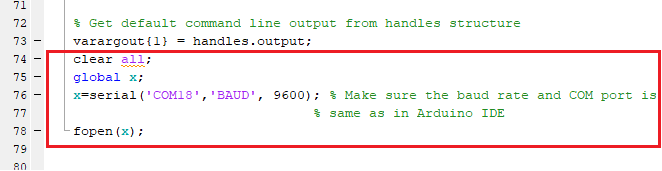where, fopen(x) is used to open the serial port for serial communication.

When you scroll down, you will see that there are two functions created for both the Buttons in the GUI. Now write the code in both the functions according to the task you want to perform on click.

In LED-ON button’s function, copy and paste the below code just before the end of the function to turn ON the LED. As you see in the below code, fprintf(x,1) is used for sending serial data from MATLAB to Arduino serial. Here we are sending ‘1’ to the Arduino serial and if you check the Arduino code, you will find that Arduino will glow the LED by making its 13th pin HIGH, when it receives ‘1’ on its serial port.

```global x;
fprintf(x,1);```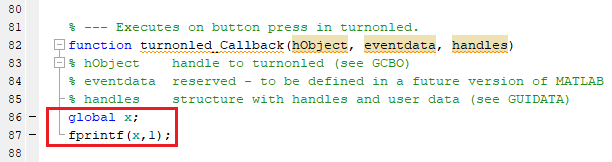In LED-OFF button’s function, copy and paste the below code just before the end of the function to turn OFF the LED. As you see in the below code, fprintf(x,0) is used for sending serial data from MATLAB to Arduino serial. In this part, we are sending ‘0’ to the Arduino serial and if you check the Arduino code, you will find that Arduino will turn off the LED by making its 13th pin LOW, when it receives ‘0’ on its serial port.

```global x;
fprintf(x,0);```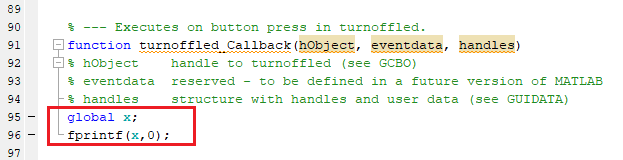After completing with MATLAB GUI coding and setup the hardware according to circuit diagram, just click on the run button to run the edited code in .m file.MATLAB may take few seconds to respond, do not click on any GUI button until MATLAB shows BUSY indication, which you can see at the left bottom corner of the screen as shown below,When everything is ready, click on LED-ON and LED-OFF button to turn ON and OFF the LED. When you press LED-ON button, 13th pin of Arduino goes HIGH and LED connected to this PIN starts glowing, and when you press LED-OFF button, 13th pin of Arduino goes LOW which makes the LED to turn off.

You can check out the video below to understand the complete process of Sending Serial Data from MATLAB to Arduino using MATLAB GUI.

Code

Arduino Code for Serial Communication between MATLAB and Arduino

int value;

void setup()
{
Serial.begin(9600);
pinMode(13, OUTPUT);
}

void loop()
{
if(Serial.available()>0)
{

if  (value == 1)
{
digitalWrite(13, HIGH);
}
if(value == 0)
{
digitalWrite(13, LOW);
}
}
}

MATLAB Code for Serial Communication using MATLAB GUI

function varargout = final(varargin)

gui_Singleton = 1;
gui_State = struct('gui_Name',       mfilename, ...
'gui_Singleton',  gui_Singleton, ...
'gui_OpeningFcn', @final_OpeningFcn, ...
'gui_OutputFcn',  @final_OutputFcn, ...
'gui_LayoutFcn',  [] , ...
'gui_Callback',   []);
if nargin && ischar(varargin{1})
gui_State.gui_Callback = str2func(varargin{1});
end

if nargout
[varargout{1:nargout}] = gui_mainfcn(gui_State, varargin{:});
else
gui_mainfcn(gui_State, varargin{:});
end

handles.output = hObject;

guidata(hObject, handles);

function varargout = final_OutputFcn(hObject, eventdata, handles)

varargout{1} = handles.output;
clear all;
global x;
x=serial('COM18','BAUD', 9600); % Make sure the baud rate and COM port is
% same as in Arduino IDE
fopen(x);

function turnonled_Callback(hObject, eventdata, handles)

global x;
fprintf(x,1);

function turnoffled_Callback(hObject, eventdata, handles)

global x;
fprintf(x,0);

Video

Tags